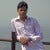# Python:- The best way to get file-type.

`filename = "abc/sample.txt"filename_split_arr = filename.split(".")print(filename_split_arr[-1])`

best practice to get file-type:-

`import magicfile_type = magic.from_file("abc/sample.txt", mime=True)print(file_type)`

python-magic is a Python interface to the libmagic file type identification library. libmagic identifies file types by checking their headers according to a predefined list of file types. This functionality is exposed to the command line by the Unix command `file`.

# Python-DB Connection in Class Wrapper.

This class uses pymysql package to do the database interaction.

`from pymysql import connectfrom pymysql.cursors import DictCursorclass Database:    def __init__(self, host_name, user_name, password, charset, port):        self._conn = connect(host=host_name, user=user_name, password=password, db=self.db, charset=charset, port=port…`

# DP Amazon Interview Quetion

A frog jumps either 1, 2 or 3 steps to go to top. In how many ways can it reach the top.

`def solution(num):  if num==1 or num==2 or num==3:    return num  return 1+solution(num-1)+solution(num-2)+solution(num-3)`

Let’s Define Dp solution using recursive

`def Dp_Solution(num):  temp=[0 for i in range(num+1)]  temp=temp=1  temp=2  temp=3  if num>3:    for i in range(4,num+1):      temp[i]=1+temp[i-1]+temp[i-2]+temp[i-3]  return temp[num]`

# Walmart Interview Question Solve using DP

Given an M X N matrix with your initial position at the top-left cell, find the number of possible unique paths to reach the bottom-right cell of the matrix from the initial position.

Note: Possible moves can be either down or right at any point in time, i.e., we can move to matrix[i+1][j] or matrix[i][j+1] from matrix[i][j].

Recursive Solution for Above Question

`def solution(m,n):    if m==1 or n==1:        return 1    return solution(m-1,n)+solution(m,n-1)print(solution(3,4))`

Using Recursive Solution let’s find Dp solution for that,

`def solution(m,n):    temp=[[0 for i in range(m)] for j in range(n)]    for i in range(m):        temp[i]=1    for j in range(n):        temp[j]=1    for i in range(1,m):        for j in range(n):            temp[i][j]=temp[i-1][j]+temp[i][j-1]    return temp[m-1][n-1]`

# DP Problem (Count distinct occurrences as a subsequence)

Given a two strings S and T, find count of distinct occurrences of T in S as a subsequence.

`# Python3 program to count number of times # S appears as a subsequence in T def findSubsequenceCount(S, T):m = len(T) n = len(S)# T can't appear as a…`

# DP Problem

Given a number n, we can divide it into only three parts n/2, n/3, and n/4 (we will consider only integer part). The task is to find the maximum sum we can make by dividing the number into three parts recursively and summing up them together.
Note: Sometimes, the maximum sum can be obtained by not dividing n.

start with the minimum solution n=0 then max sum=0 now n=1 , maxsum=1 so on. so we find a recursive solution like max(n//2+n//3+n//4,n).

`def solution(n):  dp = [0 for i in range(n+1)]  dp = 0  dp = 1  for i in range(2, n+1):    dp[i] = max(dp[int(i/2)] + dp[int(i/3)] + dp[int(i/4)], i);  return dp[n]`

# Number of subsequences of the form a^i b^j c^k

Given a string, count number of subsequences of the form a^ib^jc^k, i.e., it consists of i ’a’ characters, followed by j ’b’ characters, followed by k ’c’ characters where i >= 1, j >=1 and k >= 1.

`from collections import Counterdef solution(totalcount):  temp_ar=[0 for i in range(totalcount+1)]  temp_ar=1  for i in range(4,totalcount+1):    temp_ar[i]=temp_ar[i-1]*3  return temp_ar[totalcount]def get_totalcount(str_ar):  return solution(sum(Counter(str_ar).values()))str="abbcc"print(get_totalcount(str))`